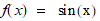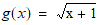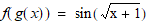# NMath User's Guide

13.1 Encapsulating Functions (.NET, C#, CSharp, VB, Visual Basic, F#)

Class encapsulates an arbitrary function, and works with other numerical classes to approximate integrals and derivatives.

NOTE—Class Polynomial extends OneVariableFunction, and provides exact methods for integration and differentiation of polynomials, as well as various convenience func­tions for creating and manipulating polynomials. This is the preferred class to use if your function is a polynomial. See Section 13.4 for more information.

Creating a Function of One Variable

A OneVariableFunction is constructed from a Func<double, double>, a function delegate that takes a single double parameter and returns a double.

For example, suppose you wish to encapsulate this function:

Code Example – C# calculus

```public double MyFunction( double x )
```
```{
```
```  return Math.Sin( x ) + Math.Pow( x, 3 ) / Math.PI;
```
```}
```

Code Example – VB calculus

```Function MyFunction(X As Double) As Double
```
```  Return Math.Sin(X) + Math.Pow(X, 3) / Math.PI
```
```End Function
```

First, create a delegate for the MyFunction() method:

Code Example – C# calculus

```var d = new Func<double, double>( MyFunction );
```

Code Example – VB calculus

```Dim D As New Func(Of Double, Double)(AddressOf MyFunction)
```

Then construct a OneVariableFunction encapsulating the delegate:

Code Example – C# calculus

```var f = new OneVariableFunction( d );
```

Code Example – VB calculus

```Dim F As New OneVariableFunction(D)
```

A Func<double, double> delegate is also implicitly converted to a OneVariableFunction. Thus:

Code Example – C# calculus

```OneVariableFunction f = d;
```

Code Example – VB calculus

```OneVariableFunction f = d;
```

Properties of Functions

A OneVariableFunction object has the following properties:

Function gets the encapsulated function delegate.

Integrator gets and sets the integration object associated with the function (see Section 13.2).

Differentiator gets and sets the differentiation object associated with the function (see Section 13.3).

Evaluating Functions

The Evaluate() method on OneVariableFunction evaluates a function at a given x-value. For instance, if f is a OneVariableFunction:

Code Example – C# calculus

```double y = f.Evaluate( Math.PI );
```

Code Example – VB calculus

```Dim Y As Double = F.Evaluate(Math.PI)
```

Evaluate() also accepts a vector of x-values, and returns a vector of y-values, such that y[i] = f( x[i] ). Thus, this code evaluates f at 100 points between 0 and 1:

Code Example – C# calculus

```var x = new DoubleVector( 100, 0, 1.0/100 );
```
```DoubleVector y = f.Evaluate( x );
```

Code Example – VB calculus

```Dim X As New DoubleVector(100, 0, 1.0 / 100.0)
```
```Dim Y As DoubleVector = F.Evaluate(X)
```

Finally, Evaluate() accepts another OneVariableFunction, and returns a new function encapsulating the composite. For example, if f encapsulates the functionand g encapsulates, you can create a new function that encapsulateslike so:

Code Example – C# calculus

```OneVariableFunction composite = f.Evaluate( g );
```

Code Example – VB calculus

```Dim Composite As OneVariableFunction = F.Evaluate(g)
```

Algebraic Manipulation of Functions

NMath provides overloaded arithmetic operators for functions with their conventional meanings for those .NET languages that support them, and equivalent named methods for those that do not. Table 10 lists the equivalent operators and methods.

 Operator Equivalent Named Method + Add() - Subtract() * Multiply() / Divide() Unary - Negate()

All binary operators and equivalent named methods work either with two functions, or with a function and a scalar. For example, this C# code uses the overloaded operators:

Code Example – C# calculus

```OneVariableFunction g = f/2;
```
```OneVariableFunction sum = f + g;
```
```OneVariableFunction neg = -f;
```

This Visual Basic code uses the equivalent named methods:

Code Example – VB calculus

```Dim G As OneVariableFunction = OneVariableFunction.Divide(F, 2)
```
```Dim Sum As OneVariableFunction = OneVariableFunction.Add(F, g)
```
```Dim Neg As OneVariableFunction = OneVariableFunction.Negate(F)
```

Finally, as a convenience, NMathFunctions provides a Pow() method that raises a function to a scalar power:

Code Example – C# calculus

```OneVariableFunction g = NMathFunctions.Pow( f, 3.5 );
```

Code Example – VB calculus

```Dim G As OneVariableFunction = NMathFunctions.Pow(F, 3.5)
```

Top

Top